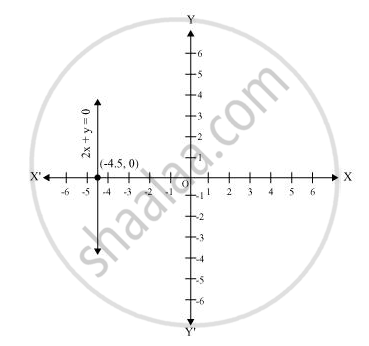# Give the geometric representations of 2x + 9 = 0 as an equation in two variables. - Mathematics

Give the geometric representations of 2x + 9 = 0 as an equation in two variables.

#### Solution

In two variables, 2x + 9 = 0 represents a straight line passing through point (−4.5, 0) and parallel to y-axis. It is a collection of all points of the plane, having their x-coordinate as 4.5.Concept: Equations of Lines Parallel to the X-axis and Y-axis
Is there an error in this question or solution?

#### APPEARS IN

NCERT Class 9 Maths
Chapter 4 Linear Equations in two Variables
Exercise 4.4 | Q 2.2 | Page 77